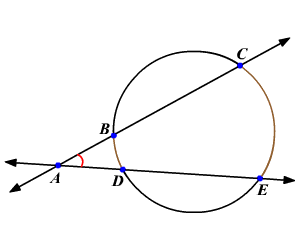# Angle of Intersecting Secants Theorem

If two lines intersect outside a circle , then the measure of an angle formed by the two lines is one half the positive difference of the measures of the intercepted arcs .In the circle, the two lines $\stackrel{↔}{AC}$ and $\stackrel{↔}{AE}$ intersect outside the circle at the point A .

$m\angle CAE=\frac{1}{2}\left(m\stackrel{⌢}{CE}-m\stackrel{⌢}{BD}\right)$

Example:

In the circle shown, if $m\stackrel{⌢}{BD}=28°$ and $m\stackrel{⌢}{CE}=88°$ , then find $m\angle CAE$ .

Substitute the angle measures.

$\begin{array}{l}m\angle CAE=\frac{1}{2}\left(m\stackrel{⌢}{CE}-m\stackrel{⌢}{BD}\right)\\ \text{\hspace{0.17em}}\text{\hspace{0.17em}}\text{\hspace{0.17em}}\text{\hspace{0.17em}}\text{\hspace{0.17em}}\text{\hspace{0.17em}}\text{\hspace{0.17em}}\text{\hspace{0.17em}}\text{\hspace{0.17em}}\text{\hspace{0.17em}}\text{\hspace{0.17em}}\text{\hspace{0.17em}}\text{\hspace{0.17em}}\text{\hspace{0.17em}}\text{\hspace{0.17em}}\text{\hspace{0.17em}}\text{\hspace{0.17em}}\text{\hspace{0.17em}}\text{\hspace{0.17em}}=\frac{1}{2}\left(88°-28°\right)\\ \text{\hspace{0.17em}}\text{\hspace{0.17em}}\text{\hspace{0.17em}}\text{\hspace{0.17em}}\text{\hspace{0.17em}}\text{\hspace{0.17em}}\text{\hspace{0.17em}}\text{\hspace{0.17em}}\text{\hspace{0.17em}}\text{\hspace{0.17em}}\text{\hspace{0.17em}}\text{\hspace{0.17em}}\text{\hspace{0.17em}}\text{\hspace{0.17em}}\text{\hspace{0.17em}}\text{\hspace{0.17em}}\text{\hspace{0.17em}}\text{\hspace{0.17em}}\text{\hspace{0.17em}}=\frac{1}{2}\left(60°\right)\\ \text{\hspace{0.17em}}\text{\hspace{0.17em}}\text{\hspace{0.17em}}\text{\hspace{0.17em}}\text{\hspace{0.17em}}\text{\hspace{0.17em}}\text{\hspace{0.17em}}\text{\hspace{0.17em}}\text{\hspace{0.17em}}\text{\hspace{0.17em}}\text{\hspace{0.17em}}\text{\hspace{0.17em}}\text{\hspace{0.17em}}\text{\hspace{0.17em}}\text{\hspace{0.17em}}\text{\hspace{0.17em}}\text{\hspace{0.17em}}\text{\hspace{0.17em}}\text{\hspace{0.17em}}=30°\end{array}$

Therefore, $m\angle CAE=30°$ .